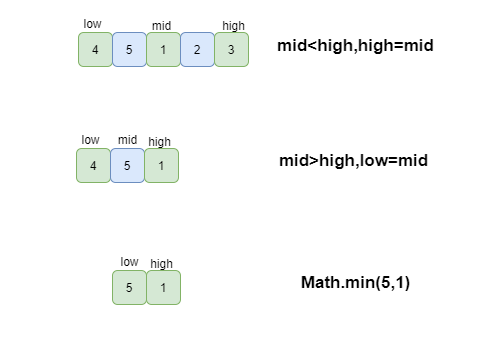[toc]

# 题目描述

NOTE：给出的所有元素都大于0，若数组大小为0，请返回0。

# 思路

• 直接遍历，当出现后面的数比前面的数小的时候，就是找到了最小的数。
• 使用二分查找，在已经排序过的数组中常用的算法。

# 代码

## 直接遍历

``````import java.util.ArrayList;
public class Solution {
public int minNumberInRotateArray(int [] array) {
if(array==null||array.length==0){
return 0;
}
if(array.length==1){
return array;
}
int max = array;
for(int i=1;i<array.length;i++){
if(array[i]>max){
max = array[i];
}
if(array[i]<max){
return array[i];
}
}
return 0;
}
}
``````

## 二分法• 取出中间元素，和最右边元素比较，如果中间元素大于最右边元素，则证明，最小值存在于中间元素到最右边元素之间的一段。如果中间元素小于最右边元素，则证明，最小值在最左边元素到中间元素之间的一段中。

``````public class Solution {
public static int minNumberInRotateArray(int[] array) {
if (array == null || array.length == 0) {
return 0;
}
if (array.length == 1) {
return array;
}
int low = 0, high = array.length - 1;
while (high - low > 1) {
int mid = (low + high) / 2;
if (array[mid] > array[high]) {
low = mid;
} else if (array[mid] < array[high]) {
high = mid;
} else {
high--;
}
}
return Math.min(array[low], array[high]);
}
}
``````

Q.E.D.